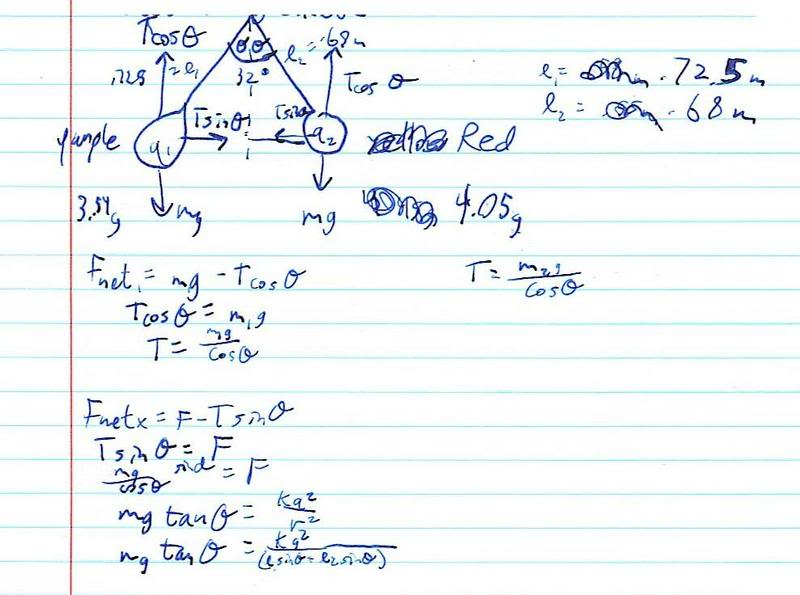# Electrostatic force and # of elementary particles

• Plasma

#### Plasma

I need to find the electrostatic force & the number of elementary particles between two charged balloons held in the center of their strings (Think of an Isosceles Triangle).

## Homework Statement

Mpurple(balloon) - .00354 kg
Mred - .00405 kg
Lpurple - .725 m
Lred - .68 m
θ = 16°
T = Tension

2. Homework Equations & The attempt at a solution

Fnet1 = Mpurple*g - Tcosθ = 0 T2 = (Mred*g)/cosθ
Tcosθ = Mpurple*g
T1 = (Mpurple*g)/cosθ

Fnetx = F-Tsinθ
Tsinθ = F
(M*g)/cosθ * sinθ = F
M*g*tanθ = (k*q²)/r²
M*g*tanθ = (k*q²)/(Lpurple*sinθ + Lred*sinθ)
(Lpurple*sinθ + Lred*sinθ)*( M*g*tanθ) = k*q²
q² = ((Lpurple*sinθ + Lred*sinθ)*( M*g*tanθ))/k
q = √(((Lpurple*sinθ + Lred*sinθ)*( M*g*tanθ))/k)
qPurple = √(((.725*sin16° + .68*sin16°)*( .00354*9.81*tan16°))/8.99x109)
qRed = √(((.725*sin16° + .68*sin16°)*( .00405*9.81*tan16°))/8.99x109)

Tpurple = (.00354 kg * 9.81m/s²)/cos16° = 0.036 N
Tred = (.00405 kg * 9.81m/s²)/cos16° = 0.041 N
qPurple = 6.54 x 10-7 C
qRed = 7.01 x 10-7 C

I don't know how I'd go about finding the Electrostatic force or the number of elementary particles. Any help is appreciated.

anyway you can post a diagram--or at least better describe the physical situation. You've done a lot of work and we would like to help..

OK, diagram coming up (If you can read my handwriting) Also, I misread the handout. I was looking for the number of elementary charges, not particles. I know how to do that, so I only need help with the electrostatic force.For electrostatic force, use the equation:

F=[1/(4*pi*e0)]*[abs(Q1)*abs(Q2)]r^2

where e0 is the permittivity of free space.

and r is the distance between the charges. Just find the distance between the balloons (which can be solved with some elementary trig) and you have your answer.

Ok, thanks.